Space, Time and Aether | OMICS International
Fluid Mechanics: Open Access

# Space, Time and Aether

David John Smith*1

Rotherham, United Kingdom

1BSc and ARCS were awarded in 1973 by the University of London, Imperial College, United Kingdom.

*Corresponding Author:
Smith DJ
Retired Engineer, Rotherham, United Kingdom
Tel: +44 7966547679
E-mail: [email protected]

Received Date: September 25, 2016; Accepted Date: October 23, 2016; Published Date: October 30, 2016

Citation: Smith DJ (2016) Space, Time and Aether. Fluid Mech Open Acc 3: 135.

Copyright: © 2016 Smith DJ. This is an open-access article distributed under the terms of the Creative Commons Attribution License, which permits unrestricted use, distribution, and reproduction in any medium, provided the original author and source are credited.

Visit for more related articles at Fluid Mechanics: Open Access

#### Abstract

At the end of the nineteenth century a luminiferous aether was considered necessary to explain electromagnetic interactions and the propagation of light waves. As this aether could not be detected it was disregarded and mathematical theories were developed to explain physical interactions without reference to the aether. Now, over a century later, it is generally acknowledged that a major part of the universe consists of Dark Energy. This article considers that dark energy could be the same entity as the elusive luminiferous aether and looks at implications for time, space and gravity. The conclusion is that results can be obtained by this method that are equivalent to those obtained according to Einstein’s theories.

#### Keywords

Aether; Dark energy; Special relativity; General relativity

#### Introduction

When Maxwell presented his Dynamical Theory of the Electromagnetic Field to the Royal Society in 1864 , an essential part of his theory was that: “there is an ethereal medium filling space and permeating bodies, capable of being set in motion and of transmitting that motion from one part to another, and of communicating that motion to gross matter so as to heat it and affect it in various ways”.

Designing experiments to detect and test properties of this medium became a high priority for physicists of the time. These experiments failed to detect any such medium either fixed in space or fixed relative to the earth.

In view of such apparently conflicting results, Einstein developed his theory of Special Relativity without reference to the aether. However, in doing so, Einstein had to redefine the familiar concepts of space and time and develop relativistic “spacetime”.

Moving forward about a hundred years to the end of the twentieth century, the existence of dark energy has been acknowledged to make up about 68% of the mass/energy density of the universe. The nature of dark energy remains a matter of speculation as it has never been directly detected but it is thought to be very homogeneous, not very dense and unlikely to be detectable in laboratory experiments.

In my book “Where There’s Dark…” , I suggest that dark energy is the same entity as the aether that is necessary according to Maxwell’s theory and show that this could lead to an alternative model of the universe that was not created as a Big Bang at a single point in space and time.

Aether as dark energy

Consider a model where dark energy (aether) behaves like a continuously variable dynamic fluid with properties of electromagnetic potential and gravitational potential. Imagine space containing dynamic pockets of this fluid, a bit like bubbles with variable but finite volumes, containing fixed quantities of energy. These pockets have mass (potential energy) and move under the influence of a gravitational field (kinetic energy) towards a gravitational focus with higher density. This motion is countered by thermodynamic pressure within the fluid (kinetic energy), which pushes mass away from higher density. Thus, constantly changing opposing forces result in thermodynamic motion similar to that described for a gas by Kinetic Theory but with pockets of aether rather than gas molecules. At the surface of the Earth, the gravitational force is directed towards the centre of gravity (Figure 1).

Figure 1: Depiction of the Aether in the vicinity of the Earth with spherical symmetry centred on the centre of gravity.

According to Maxwell’s theory , the speed of light is,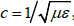, where μ is magnetic permeability and ε is electric permittivity of the aether. If these electromagnetic properties are constant and independent of density, then the speed of light is always the same in any direction irrespective of any motion of the source. This is consistent with the results of experiments that failed to detect the aether and is consistent with Einstein’s Special Theory of Relativity [3,4].

Waves in moving frames of reference

Consider two reference frames in the vicinity of the surface of the Earth, one “stationary” frame and one “moving” frame. Each frame contains aether with identical properties. In both frames the aether is subject to a gravitational force in the vertical direction towards the centre of gravity of the Earth. This force is countered by thermodynamic pressure and there is also balanced thermodynamic pressure in the horizontal directions. If the relative movement between the frames is horizontal then the effect of gravity will be the same in both frames and can be neglected for this scenario.

The electromagnetic properties of the aether, and therefore the speed of light, are the same whether determined within the stationary frame or in the moving frame. Waves emitted within the stationary frame will be observed as spherical waves propagating at velocity, c, within the stationary frame and waves emitted within the moving frame will be observed as spherical waves propagating at velocity, c, within the moving frame (Figure 2).

We now consider waves emitted in a stationary frame being observed in a moving frame and vice versa. The Doppler Effect causes a shift in frequency and wavelength when observed with a relative velocity. The waves are compressed when the source is moving towards the observer and the waves are stretched when the source is moving away from the observer (Figure 3).

If we consider waves emitted at wavelength λE, frequency fE, in the stationary frame being observed in a frame moving away from the source with a relative velocity v, the waves are observed with a stretched wavelength λO given by: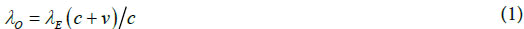Using the relationships, λE fE = c and λO fO = c, the observed frequency is given by: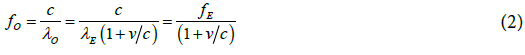If we then consider waves emitted in the moving frame at wavelength λE, frequency fE being observed in the stationary frame as the source moves away, the waves are emitted, at velocity c relative to the source but this is velocity c – v relative to the observer. The waves are then observed at velocity c with a reduced frequency fO: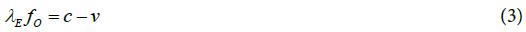The observed frequency is given by: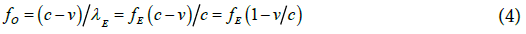Both frames are identical with the only motion being relative to the other frame. There is no way of determining which frame is moving and which frame is stationary. It is only the relative velocity between the two frames that is significant and equations (2) and (4) are both equally valid. Multiplying the two together gives: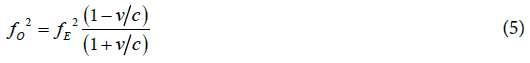or;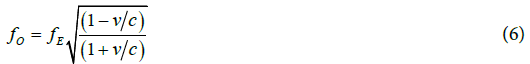and;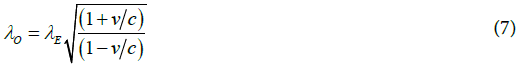This is the same result as given by Einstein’s Special Theory of Relativity .

If waves emitted in a stationary frame are received in a moving frame and then reflected back from the moving frame to the stationary frame, the frequency and wavelength is shifted again when observed back in the stationary frame (Figure 4).

The waves are received in the moving frame at wavelength λM, frequency fM.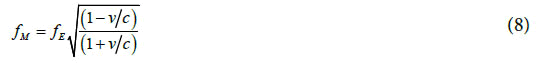The waves are reflected back to the stationary frame and then observed at wavelength λO, frequency fO.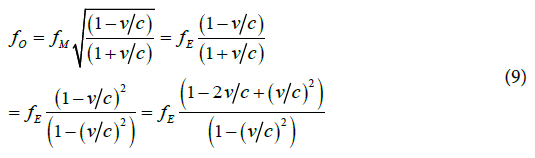For v << c, the (v/c)2 terms can be neglected, giving: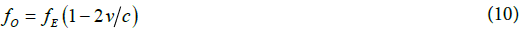and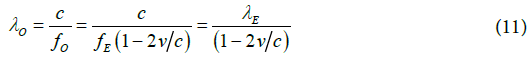In this way the velocity, v, can be determined from the change in frequency δf = fE – fO relative to the initial frequency as: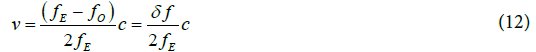This effect is used by Doppler radar systems to measure the speed of a moving object and is put to particular good use in the application of motor vehicle speed cameras.

If a source is moving at an angle relative to an observer it is the component of velocity that is directed from source to observer that determines the observed frequency rather than the absolute velocity of the source.

Determination of time

When Einstein produced his Special Theory of Relativity in 1905, he did not have the advantage of modern technology that we use today. The familiar method of determining time was by reference to mechanical clocks. Synchronization of remote clocks was a problem for Einstein. A hundred years later, clocks are continuously synchronized with the internet, GPS systems, air traffic control and space travel all being totally reliant on the ability to seamlessly synchronize clocks.

Einstein described a method for synchronizing clocks in a stationary system by considering two clocks, A and B with corresponding “A time” and “B time”. He described the process as follows:

Let A send a signal to B at the speed of light starting at time tA and let this be reflected from B at time tB such that it is received back by A at time tA’. Then the two clocks are synchronized if: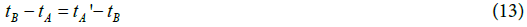Any number of clocks can be synchronized in this way. If AB is the distance from A to B then the speed of light is: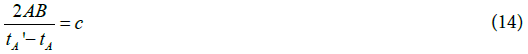Where, c is the speed of light and the time defined in this way is “the time of the stationary system”.

Einstein then considered the same process in a moving system and concluded that space and time in the moving system differed from space and time in the stationary system.

However, by examining the process and taking account of the different path lengths we can show that it is possible to use Einstein’s method to synchronize clocks in a moving system with clocks in a stationary system (Figure 5).

Various scenarios can be examined as to whether the wave is emitted or observed in the stationary or moving system but for each scenario it can be shown that the clocks can be synchronised by Einstein’s rule. For example if the wave is emitted and observed in the stationary system:

The wave travels from A to B at speed c, frequency f, path length A to B1, elapsed time tB - tA.

The wave travels from B to A at speed c, frequency f(1-v/c)/(1+v/c), path length B1 to A2, elapsed time tA’ - tB. Note that there is a double frequency shift due to reflection in the moving frame as in equation (9).

The wave travels from A2 to A0 elapsed time tA’’ - tA’.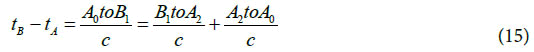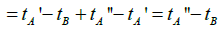The clocks are still synchronized according to Einstein’s rule as tB - tA = tA’’ - tB. Also by measuring the relative change in frequency (δf/f) between the outgoing wave and the incoming wave an observer in the stationary system can determine the relative velocity by equation (12) (v = c × δf /2f, for v << c). In this way, any clock located on the surface of the earth can be synchronized with any clock on a moving aircraft and any clock on a moving spacecraft.

We can conclude that elapsed time measured in the moving system is the same as elapsed time measured in the stationary system. However, there is a shift in frequency according to the relative velocity between stationary and moving systems. If time periods are measured as the inverse of frequency then there is a change in time periods (τ) as: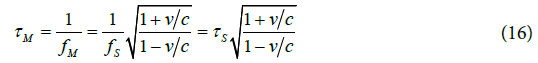Where τM and fM are measured in a frame moving with velocity v away from the frame with τS and fS. This result is the same as that determined by Einstein, although the interpretation is different. We determine a shift in measured time periods rather than a difference in elapsed time.

Space and density

The above section shows that the transformation of time coordinates used by Einstein is valid mathematically but it is not necessary. Einstein uses a transformation of time coordinates then derives transformations of space coordinates, which are again valid mathematically but not necessary.

The concept of measurement against a defined coordinate system is a human invention. Physical processes don’t depend on coordinate systems. If a source emits an electromagnetic wave it is a consequence of internal properties of the source and external properties of its surroundings with no reference to any coordinate system and this is the case for any other physical interaction.

Consider a source emitting a circularly polarized electromagnetic wave. The normal to the wavefront is a straight line path. At any point along this path the wave can be considered in terms of electromagnetic field vectors rotating at angular velocity ω (Figure 6).

If the wave is emitted with a frequency fE = ω/2π and an observer is moving towards the source with a relative velocity of -v, then the observed frequency is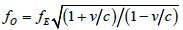. If the observer is moving away from the source with a relative velocity of +v, then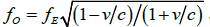. If the observer is rotating relative to the source with an angular velocity of +ωR, then the observed frequency is fO = fE + ωR/2π. If the relative motion between source and observer has linear velocity, v, and angular velocity, ωR, then the observed frequency is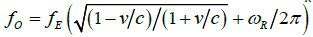An observer could quantify these differences with reference to any coordinate system whether fixed relative to the source, the observer or any other reference point providing that the relative linear and angular velocities could be determined. The results of such an analysis would be identical to results determined using Einstein’s methods. A change in frequency is the inverse of a change in time interval and a difference in path length for a light signal can be interpreted as length contraction.

One of the most significant conclusions from Einstein’s Special Theory of Relativity was that inert mass is nothing more or less than energy and this can be represented by the equation: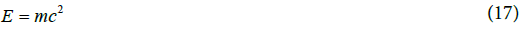This could equally be written using properties of the aether without reference to the speed of light: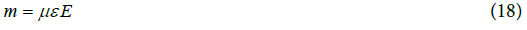Where E is energy and m is mass. The electromagnetic properties of the aether are constant and independent of density and are unaffected by any motion through or within the aether.

From his General Theory of Relativity , Einstein concluded that “Euclidean geometry does not hold even to a first approximation in the gravitational field” and that “the rate of a unit clock, which is arranged to be at rest in a static gravitational field… goes more slowly if it is set up in the neighbourhood of ponderable masses”.

If we consider Einstein’s transformation of coordinates as being mathematically valid but not physically necessary, then there could be an alternative interpretation of Einstein’s conclusions. If we consider a volume of aether as a quantity of dark energy with a known mass, then if this quantity of energy is in the presence of a gravitation field, a reduction in volume by transformation of coordinates is equivalent to an increase in density as density = mass/volume. Einstein’s transformation of coordinates in relativistic spacetime is then equivalent to an increase in density of the aether in normal Euclidean space.

Gravity as a density gradient in the aether

A gravitational field results in a density gradient within the aether and, conversely, a density gradient within the aether results in a gravitational field. Classical gravitational theory, based on Newton’s inverse-square law, gives: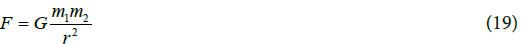Where F is force, m1 and m2 are masses of two interacting objects, r is the distance between the centres of the masses and G is the gravitational constant.

Although Newton's theory has been superseded by Einstein's theory, gravitational calculations are still made using Newton's equation. According to classical theory, gravitational potential is work stored in a force field as potential energy. In the absence of any restraining force, a difference in gravitational potential results in an acceleration generally denoted as “g”, also described as a gravitational field.

This gravitational field is a conservative vector field that can be described as the gradient of a scalar potential, φ: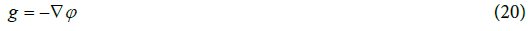Where, ∇ is the three dimensional gradient operator. The gravitational field due to a spherically symmetric mass is a function of only one variable, r, the distance from the centre of mass and therefore: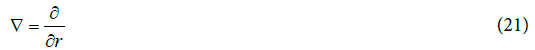If we consider that gravitational potential, φ, is proportional to aether density, then: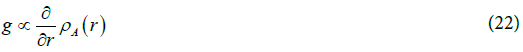Where, ρA(r) is the density of the aether at a distance r from the centre of a spherically symmetric mass, M. If we use the electromagnetic properties of the aether to match dimensions in SI units and measure the density of the aether ρA(r) relative to the average density of dark energy, denoted as ρΛ, we get: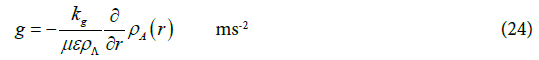Where, kg is an unknown numerical constant. The negative sign indicates that g reduces as r increases.

This can be compared with Newton’s law where: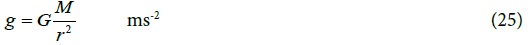giving;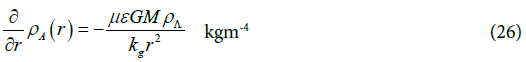Integrating this gives the relative increase in density of the aether at a distance, r, from a spherically symmetrical mass, M: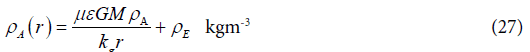Where, ρE is a constant of integration and is the background density of the aether including contributions from other external gravitational fields.

#### Conclusions

By considering that dark energy is the same entity as the elusive luminiferous aether that was sought a hundred years ago, results can be obtained that are equivalent to those obtained according to Einstein’s theories.

The transformation of time described by Einstein is equivalent to the inverse of a transformation of frequency by the Doppler Effect.

The transformation of space described by Einstein is equivalent to an increase in density of the aether.

A gravitational field is a density gradient within the aether and a density gradient within the aether is a gravitational field.

#### References

Select your language of interest to view the total content in your interested language

### Article Usage

• Total views: 10663
• [From(publication date):
October-2016 - Jan 18, 2020]
• Breakdown by view type
• HTML page views : 10481Can't read the image? click here to refresh